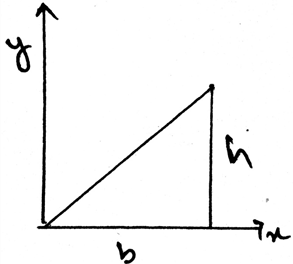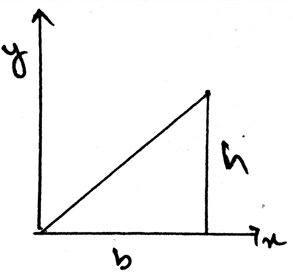# Test: Product Of Inertia For An Area

## 16 Questions MCQ Test Engineering Mechanics | Test: Product Of Inertia For An Area

Description
This mock test of Test: Product Of Inertia For An Area for Mechanical Engineering helps you for every Mechanical Engineering entrance exam. This contains 16 Multiple Choice Questions for Mechanical Engineering Test: Product Of Inertia For An Area (mcq) to study with solutions a complete question bank. The solved questions answers in this Test: Product Of Inertia For An Area quiz give you a good mix of easy questions and tough questions. Mechanical Engineering students definitely take this Test: Product Of Inertia For An Area exercise for a better result in the exam. You can find other Test: Product Of Inertia For An Area extra questions, long questions & short questions for Mechanical Engineering on EduRev as well by searching above.
QUESTION: 1

### The product of Inertia for an area is required so as to _____________

Solution:

The product is required as to know what is the maximum and the minimum moment of inertia of an area. This data is required as to design the structure of the body. This means that the designing of the body is majorly done by the help of the determination of the product of the moment of Inertia.

QUESTION: 2

### Determine the product of inertia for the triangle in the given figure.Solution:

The moment of inertia of the section is the integration of the square of the distance of the centroid and the del area along the whole area of the structure. This is having much significance in the various fields in the engineering sector. The main types are the ‘I’ section structures which are being much used.

QUESTION: 3

### Moment of Inertia is the integration of the square of the distance of the centroid and the del area along the whole area of the structure and after these calculations we multiply the moment of areas.

Solution:

The moment of inertia of the section is the integration of the square of the distance of the centroid and the del area along the whole area of the structure. This is having much significance in the various fields in the engineering sector. The main types are the ‘I’ section structures which are being much used.

QUESTION: 4

There is perpendicular axis theorem for the area, and it is can be used to determine the product of moment of inertia.

Solution:

There is no perpendicular axis theorem for the area. In spite there is the theorem as parallel axis for any area. Thus we have the theorem which is used to add the two mutually perpendicular moment of inertias.

QUESTION: 5

What is parallel axis theorem and to whom it is applied so that it can give the product of inertia of an area?

Solution:

Parallel axis for any area is used to add the two mutually perpendicular moment of inertias for areas. It gives a moment of inertia perpendicular to the surface of the body. That is the moment of inertia perpendicular to the surface in considerance.

QUESTION: 6

The product of moment of inertia is the sum of _____________ and _________________

Solution:

The product of moment of inertia is required as to design the structure of the body. This means that the designing of the body is majorly done by the help of the determination of the product of the moment of Inertia.

QUESTION: 7

The distance in the parallel axis theorem for the use in the determination of the product of moment of inertia is multiplied by:

Solution:

Parallel axis for any area is used to add the two mutually perpendicular moment of inertias for areas. It gives a moment of inertia perpendicular to the surface of the body. And uses the square of the distance from the axis of rotation multiplied by the area.

QUESTION: 8

One of the use of the centre of mass or centroid is as in the determination of the product of moment of inertia is that the net force acts at the ___________ of the loading body.

Solution:

In the moment of inertia calculations we see that the net force acts at the centroid of the loading body. That is if the loading system is in the form of the triangle then the at the distance 2 by 3 of the base the net force of the loading will act. And the load will be half the area of the loading.

QUESTION: 9

The product of Inertia for an area is helpful so as to _____________

Solution:

The product is required as to know what is the maximum and the minimum moment of inertia of an area. This data is required as to design the structure of the body. This means that the designing of the body is majorly done by the help of the determination of the product of the moment of Inertia.

QUESTION: 10

Determine the del product of inertia for the triangle in the given figure used for the calculations.Solution:

The moment of inertia of the section is the integration of the square of the distance of the centroid and the del area along the whole area of the structure. This is having much significance in the various fields in the engineering sector. The main types are the ‘I’ section structures which are being much used.

QUESTION: 11

If the non-Uniform loading is of the type of parabola then for calculating the product of moment of inertia for areas?

Solution:

The net force will act at the centroid of the parabola. Whether it be a parabola or the cubic curve the centroid is the only point at which the net force act. Force can’t be acted horizontally if the loading is vertical. Hence whatever be the shape of the loading, the centroid is the point of action of net force. Thus the use of centroid.

QUESTION: 12

If any external force also is applied on the structure and we are determining the product of moment of inertia then what should we consider?

Solution:

The external forces are treated differently. They are not added by the force of the distributed loading. That is the force not only acts at the centroid always. It can be shifted also. Depending on the external forces. Thus the use of centroid or centre of mass.

QUESTION: 13

The body is sometimes acted by two or three force members and we need to find the product of moment of inertia for the same. The difference between the two and the three force members is:

Solution:

The definition of the two force member only defines that the forces are being acted on the two points on the body. So does is the definition of the three forces members. The points of action of the three forces are three.

QUESTION: 14

Whenever the distributed loading acts perpendicular to an area its intensity varies __________ for the determination of the product of moment of inertia.

Solution:

The load intensity is varying linearly in the structures. Thus the intensity is not varying parabolically nor is it cubically. It cannot be a vector also. Thus the intensity is linearly varied.

QUESTION: 15

The calculation of the product of moment of the body due to the loadings involve a quantity called ____________

Solution:

The calculation of the moment of the body due to the loadings involve a quantity called moment of inertia. This is having much significance in the various fields in the engineering sector. The main types are the ‘I’ section structures which are being much used.

QUESTION: 16

Whenever the distributed loading acts perpendicular to an area its intensity varies __________ for the determination of the product of moment of inertia.

Solution:

The load intensity is varying linearly in the structures. Thus the intensity is not varying parabolically nor is it cubically. It cannot be a vector also. Thus the intensity is linearly varied.